# Primal-Dual Proximal Splitting¶

$\newcommand{\dotp}{\langle #1, #2 \rangle}$ $\newcommand{\enscond}{\lbrace #1, #2 \rbrace}$ $\newcommand{\pd}{ \frac{ \partial #1}{\partial #2} }$ $\newcommand{\umin}{\underset{#1}{\min}\;}$ $\newcommand{\umax}{\underset{#1}{\max}\;}$ $\newcommand{\umin}{\underset{#1}{\min}\;}$ $\newcommand{\uargmin}{\underset{#1}{argmin}\;}$ $\newcommand{\norm}{\|#1\|}$ $\newcommand{\abs}{\left|#1\right|}$ $\newcommand{\choice}{ \left\{ \begin{array}{l} #1 \end{array} \right. }$ $\newcommand{\pa}{\left(#1\right)}$ $\newcommand{\diag}{{diag}\left( #1 \right)}$ $\newcommand{\qandq}{\quad\text{and}\quad}$ $\newcommand{\qwhereq}{\quad\text{where}\quad}$ $\newcommand{\qifq}{ \quad \text{if} \quad }$ $\newcommand{\qarrq}{ \quad \Longrightarrow \quad }$ $\newcommand{\ZZ}{\mathbb{Z}}$ $\newcommand{\CC}{\mathbb{C}}$ $\newcommand{\RR}{\mathbb{R}}$ $\newcommand{\EE}{\mathbb{E}}$ $\newcommand{\Zz}{\mathcal{Z}}$ $\newcommand{\Ww}{\mathcal{W}}$ $\newcommand{\Vv}{\mathcal{V}}$ $\newcommand{\Nn}{\mathcal{N}}$ $\newcommand{\NN}{\mathcal{N}}$ $\newcommand{\Hh}{\mathcal{H}}$ $\newcommand{\Bb}{\mathcal{B}}$ $\newcommand{\Ee}{\mathcal{E}}$ $\newcommand{\Cc}{\mathcal{C}}$ $\newcommand{\Gg}{\mathcal{G}}$ $\newcommand{\Ss}{\mathcal{S}}$ $\newcommand{\Pp}{\mathcal{P}}$ $\newcommand{\Ff}{\mathcal{F}}$ $\newcommand{\Xx}{\mathcal{X}}$ $\newcommand{\Mm}{\mathcal{M}}$ $\newcommand{\Ii}{\mathcal{I}}$ $\newcommand{\Dd}{\mathcal{D}}$ $\newcommand{\Ll}{\mathcal{L}}$ $\newcommand{\Tt}{\mathcal{T}}$ $\newcommand{\si}{\sigma}$ $\newcommand{\al}{\alpha}$ $\newcommand{\la}{\lambda}$ $\newcommand{\ga}{\gamma}$ $\newcommand{\Ga}{\Gamma}$ $\newcommand{\La}{\Lambda}$ $\newcommand{\si}{\sigma}$ $\newcommand{\Si}{\Sigma}$ $\newcommand{\be}{\beta}$ $\newcommand{\de}{\delta}$ $\newcommand{\De}{\Delta}$ $\newcommand{\phi}{\varphi}$ $\newcommand{\th}{\theta}$ $\newcommand{\om}{\omega}$ $\newcommand{\Om}{\Omega}$

This tour explores a primal-dual proximal splitting algorithm, with application to imaging problems.

In :
library(imager)
library(pracma)
library(plot3D)

options(warn=-1) # turns off warnings, to turn on: "options(warn=0)"

# Importing the libraries
for (f in list.files(path="nt_toolbox/toolbox_general/", pattern="*.R")) {
source(paste("nt_toolbox/toolbox_general/", f, sep=""))
}
for (f in list.files(path="nt_toolbox/toolbox_signal/", pattern="*.R")) {
source(paste("nt_toolbox/toolbox_signal/", f, sep=""))
}


## Convex Optimization with a Primal-Dual Scheme¶

In this tour we use the primal-dual algorithm detailed in:

Antonin Chambolle and Thomas Pock A First-order primal-dual algorithm for convex problems with application to imaging, Journal of Mathematical Imaging and Vision, Volume 40, Number 1 (2011), 120-145

One should note that there exist many other primal-dual schemes.

We consider general optimization problems of the form $$\umin{f} F(K(f)) + G(f)$$ where $F$ and $G$ are convex functions and $K : f \mapsto K(f)$ is a linear operator.

For the primal-dual algorithm to be applicable, one should be able to compute the proximal mapping of $F$ and $G$, defined as: $$\text{Prox}_{\gamma F}(x) = \uargmin{y} \frac{1}{2}\norm{x-y}^2 + \ga F(y)$$ (the same definition applies also for $G$).

The algorithm reads: $$g_{k+1} = \text{Prox}_{\sigma F^*}( g_k + \sigma K(\tilde f_k))$$ $$f_{k+1} = \text{Prox}_{\tau G}( f_k-\tau K^*(g_k) )$$ $$\tilde f_{k+1} = f_{k+1} + \theta (f_{k+1} - f_k)$$

The dual functional is defined as $$F^*(y) = \umax{x} \dotp{x}{y}-F(x).$$ Note that being able to compute the proximal mapping of $F$ is equivalent to being able to compute the proximal mapping of $F^*$, thanks to Moreau's identity: $$x = \text{Prox}_{\tau F^*}(x) + \tau \text{Prox}_{F/\tau}(x/\tau)$$

It can be shown that in the case $\theta=1$, if $\sigma \tau \norm{K}^2<1$, then $f_k$ converges to a minimizer of the original minimization of $F(K(f)) + G(f)$.

More general primal-dual schemes have been developped, see for instance

L. Condat, A primal-dual splitting method for convex optimization involving Lipschitzian, proximable and linear composite terms, J. Optimization Theory and Applications, 2013, in press.

## Inpainting Problem¶

We consider a linear imaging operator $\Phi : f \mapsto \Phi(f)$ that maps high resolution images to low dimensional observations. Here we consider a pixel masking operator, that is diagonal over the spacial domain.

In :
name = "nt_toolbox/data/lena.png"
n = 256
f0 = rescale(crop(f0, n / 2))


Display it.

In :
options(repr.plot.width=5, repr.plot.height=5)
f0 = f0[,]
imageplot(f0)We consider here the inpainting problem. This simply corresponds to a masking operator.

Load a random mask $\La$.

In :
rho = .8
Lambda = rand(n / 2, n / 2) > rho


Masking operator $\Phi$.

In :
Phi = function(f){f * Lambda}


Compute the observations $y=\Phi f_0$.

In :
y = Phi(f0)


Display it.

In :
imageplot(y)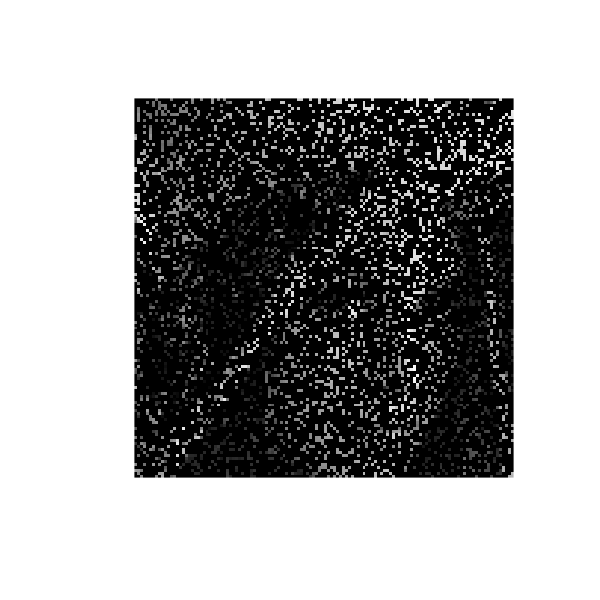## Total Variation Regularization under Constraints¶

We want to solve the noiseless inverse problem $y=\Phi f$ using a total variation regularization: $$\umin{ y=\Phi f } \norm{\nabla f}_1$$

This can be recasted as the minimization of $F(K(f)) + G(f)$ by introducing $$G(f)=i_H(f), \quad F(u)=\norm{u}_1 \qandq K=\nabla,$$ where $H = \enscond{x}{\Phi(x)=y}$ is an affine space, and $i_H$ is the indicator function $$i_H(x) = \choice{ 0 \qifq x \in H, \\ +\infty \qifq x \notin H. }$$

Shorcut for the operators.

In :
K  = function(f){grad_3(f)}
KS = function(u){-div_2(u)}


Shortcut for the TV norm.

In :
Amplitude = function(u){sqrt(u[,,1]^2 + u[,,2]^2)}
F = function(u){sum(sum(Amplitude(u)))}


The proximal operator of the vectorial $\ell^1$ norm reads $$\text{Prox}_{\lambda F}(u) = \max\pa{0,1-\frac{\la}{\norm{u_k}}} u_k$$

In :
ProxF = function(u,lambda)
{
return(pmax(0,1 - lambda / array(c(Amplitude(u), Amplitude(u)), dim=c(dim(u),dim(u),2))) * u)
}


Display the thresholding on the vertical component of the vector.

In :
t = -linspace(-2,2, 201)
Y = meshgrid(t,t)$Y X = meshgrid(t,t)$X
U = array(c(X,Y), dim=c(201, 201, 2))
V = ProxF(U,1.)

persp3D(X, Y, V[,,1], theta=30, phi=50, ticktype="detailed")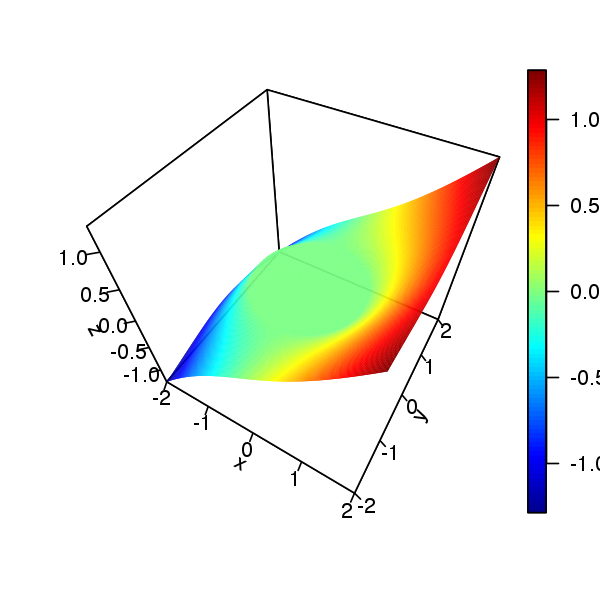For any 1-homogeneous convex functional, the dual function is the indicator of a convex set. For the $\ell^1$ norm, it is the indicator of the $\ell^\infty$ ball $$F^* = i_{\norm{\cdot}_\infty \leq 1} \qwhereq \norm{u}_\infty = \umax{i} \norm{u_i}.$$

The proximal operator of the dual function is hence a projector (and it does not depend on $\sigma$ ) $$\text{Prox}_{\sigma F^*}(u) = \text{Proj}_{\norm{\cdot}_\infty \leq 1}(u).$$

A simple way to compute the proximal operator of the dual function $F^*$, we make use of Moreau's identity: $$x = \text{Prox}_{\tau F^*}(x) + \tau \text{Prox}_{F/\tau}(x/\tau)$$

In :
ProxFS = function(y,sigma){y - sigma * ProxF(y / sigma, 1. / sigma)}


Display this dual proximal on the vertical component of the vector.

In :
V = ProxFS(U,1)
persp3D(X, Y, V[,,1], theta=20, phi=50, ticktype="detailed")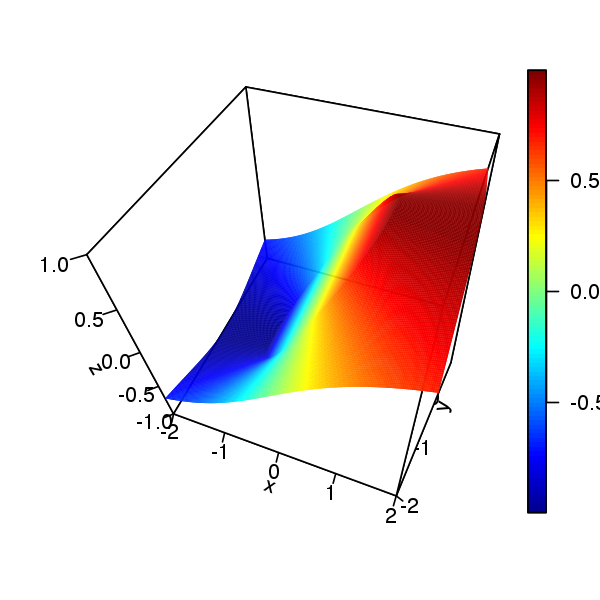The proximal operator of $G = i_H$ is the projector on $H$. In our case, since $\Phi$ is a diagonal so that the projection is simple to compute $$\text{Prox}_{\tau G}(f) = \text{Proj}_{H}(f) = f + \Phi(y - \Phi(f))$$

In :
ProxG = function(f,tau){f + Phi(y - Phi(f))}


## Primal-dual Total Variation Regularization Algorithm¶

Now we can apply the primal dual scheme to the TV regularization problem.

We set parameters for the algorithm. Note that in our case, $L=\norm{K}^2=8$. One should has $L \sigma \tau < 1$.

In :
L = 8
sigma = 10
tau = .9/(L * sigma)
theta = 1


Initialization, here |f| stands for the current iterate $f_k$, |g| for $g_k$ and |f1| for $\tilde f_k$.

In :
f = y
g = K(y) * 0
f1 = f


Example of one iterations.

In :
fold = f
g = ProxFS(g + sigma * K(f1), sigma)
f = ProxG(f - tau * KS(g), tau)
f1 = f + theta * (f - fold)


Exercise 1

Implement the primal-dual algorithm. Monitor the evolution of the TV energy $F(K(f_k))$ during the iterations. Note that one always has $f_k \in H$ so that the iterates satisfies the constraints.

In :
source("nt_solutions/optim_5_primal_dual/exo1.R")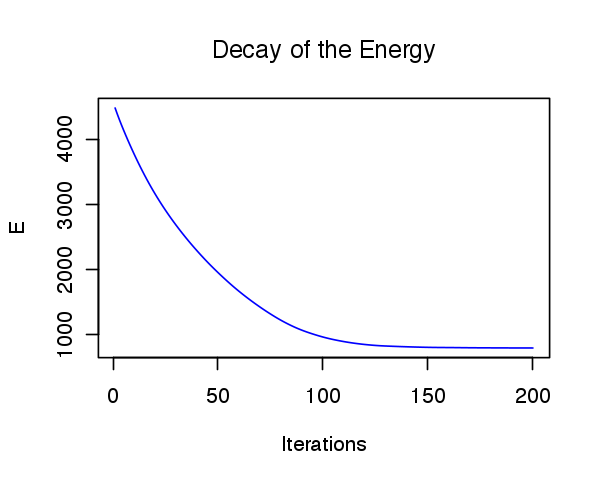In :
# Insert code here


Display inpainted image.

In :
imageplot(f)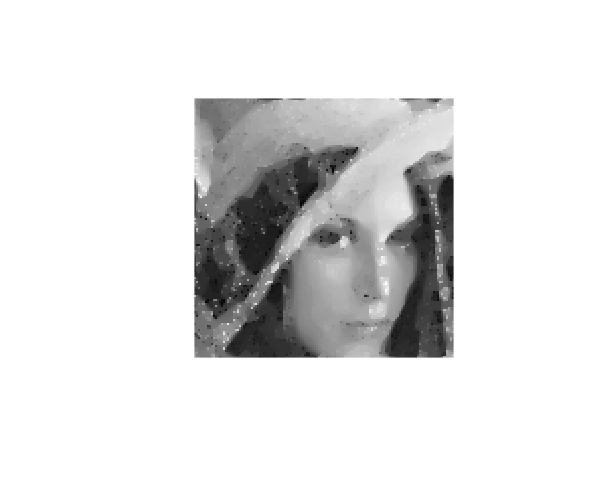Exercise 2

Use the primal dual scheme to perform regularization in the presence of noise $$\umin{\norm{y-\Phi(f)} \leq \epsilon} \norm{\nabla f}_1.$$

In :
source("nt_solutions/optim_5_primal_dual/exo2.R")

In :
# Solution not available


## Inpainting Large Missing Regions¶

It is possible to consider a more challening problem of inpainting large missing regions.

To emphasis the effect of the TV functional, we use a simple geometric image.

In :
n = 64
t = linspace(-1,1,n)
Y = meshgrid(t,t)$Y X = meshgrid(t,t)$X
f0 = (pmax(abs(X),abs(Y) ) < radius) * 1.0


We remove the central part of the image.

In :
a = 4
Lambda = matrix(1, n, n)
Lambda[((n/2) - a):((n/2) + a),] = 0


Display.

In :
imageplot(f0, 'Original', c(1,2,1))
imageplot(Phi(f0), 'Damaged', c(1,2,2))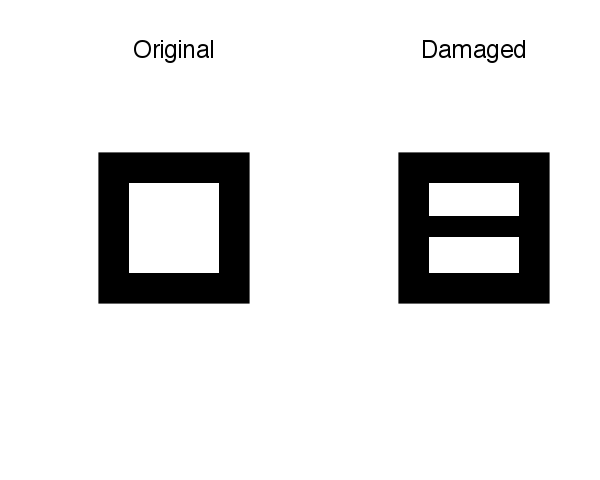Exercise 3

Display the evolution of the inpainting process.

In :
source("nt_solutions/optim_5_primal_dual/exo3.R")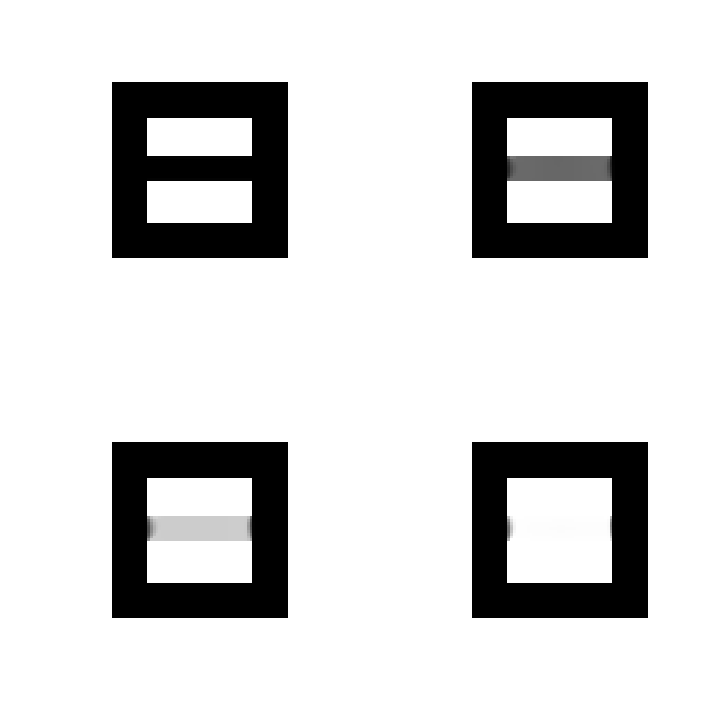In :
# Insert code here